Workers

9 workers dig a canal 120 meters long for eight hours. For how long would be dig five workers canal 200 meters long?

Result

t =  24 h

Solution:Leave us a comment of this math problem and its solution (i.e. if it is still somewhat unclear...):Be the first to comment!To solve this verbal math problem are needed these knowledge from mathematics:

Do you have a linear equation or system of equations and looking for its solution? Or do you have quadratic equation?

Next similar math problems:

1. Workers 2Worker dug the trench for 7 hours, another worker for 10 hours. They recruited to work even one worker more. Digging should be done for 2 hours. For how long would dig third worker alone?
2. Six workersSix workers planned to repair the roof in 5 days. After two days, two workers get sick. How long will the remaining workers complete the roof at the same rate of work?
3. Sprayers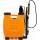The vineyard can be sprayed first sprayer in 5 hours and with the second in 8 hours. How long would it take if we use sprayers both at once?
4. Five tractors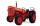Five tractors plow field in 4.8 hours. How long plow this field if after 1.5 hours of work two tractors went to a different field?
5. Welders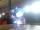Welder do the job for six hours, the second one for 8 hours. How long do the job third welder if all together can do job in 2 hours?
6. Apprentice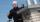Worker and apprentice execute the work for 6 hours. Worker performs himself in 10 hours. How long do the work of an apprentice?
7. Painters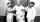One painter could paint a school for 7 days. The second one for 6 days. It was necessary to paint the school in two days. Next painter was invited and all worked together. How long would this painter painted the school itself?
8. Four painters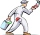The company sent four painters to paint the school. They should be done in 12 days. After three days, one painter got ill. How long will the remaining painters paint the school?
9. Hectoliters of waterThe pool has a total of 126 hectoliters of water. The first pump draws 2.1 liters of water per second. A second pump pumps 3.5 liters of water per second. How long will it take both pumps to drain four-fifths of the water at the same time?
10. The tank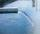The tank had 9 inflows to be filled in 21 days. After 9 days, 3 trips out. How many days did the remaining 6 tributaries fill the tank?
11. Tonic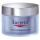One package tonic consume Mom 12 weeks and daughter for 14 weeks. 4 weeks they use the same cream together and then only daughter. How many weeks endured tonic to daughter then?
12. Liquid soap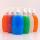Liquid soap lasted two weeks to one member of the family. How many days will last, if it use: a) two members b) 4 members c) seven members?
13. Fifth of the numberThe fifth of the number is by 24 less than that number. What is the number?
14. Unknown numberIdentify unknown number which 1/5 is 40 greater than one tenth of that number.
15. Reciprocal equationDetermine the root of the equation: 9/x-7/x=1
16. Simply equationSolve this equation for x: ?
17. BicycleThe bicycle pedal gear has 36 teeth, the rear gear wheel has 10 teeth. How many times turns rear wheel, when pedal wheel turns 120x?